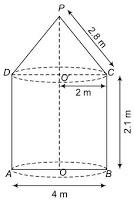# A tent is in the shape of a cylinder surmounted by a conical top. If the height and diameter of the cylindrical part are 2.1 m and 4 m respectively, and the slant height of the top is 2.8 m, find the area of the canvas used for making the tent. Also, find the cost of the canvas of the tent at the rate of Rs 500 per m2. (Note that the base of the tent will not be covered with canvas.)Given

Diameter = 4 m

Slant height of the cone (l) = 2.8 m

Radius of the cone (r) = Radius of cylinder = 4/2 = 2 m

Height of the cylinder (h) = 2.1 m

Find out

We have to find out

(i) The area of the canvas used for making the tent.

(ii) The cost of the canvas of the tent at the rate of Rs 500 per m2.

Solution

Area of canvas =C.S.A of cone+ C.S.A of cylinder

=πrl+2πrh

=πr(l+2h)

=22/7(2.8+2*2.1)

=44*(2.8+4.2)/7

=44*7/7

=44m2

ii) cost of canvas=Area*rate

=44m2*Rs. 500/m2

=Rs. 22,000

The area of the cone =44m2

Cost of Canvas = Rs 22,000(1)(0)